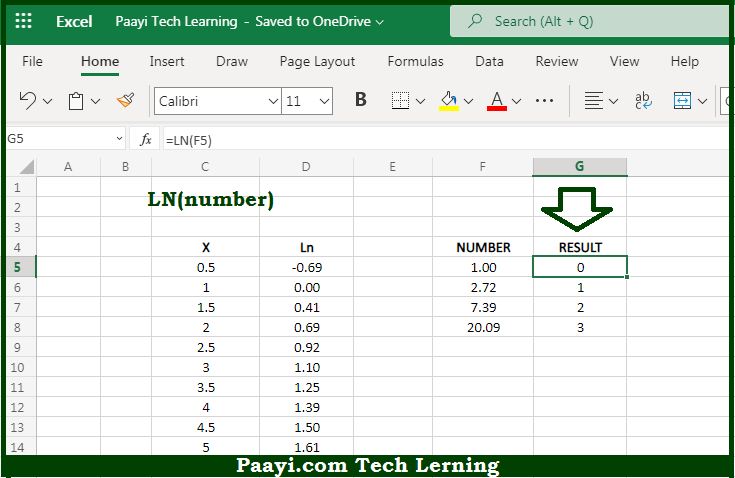# Learn How to Use Microsoft Excel LN Function

Written by | 0 Comments | 628 Views

In this article, you will learn how to use the Microsoft Excel LN function and its prime function in Microsoft Excel. You will also get to know the Microsoft Excel LN function return value and syntax with the help of some examples.

Microsoft Excel LN Function

The main function of the Microsoft Excel LN function is to get the natural logarithm of the number. It should be noted that the natural logarithm can be defined as the area under hyperbola in an "x" and "y" axis graph. The LN function is used in applications related the compound interest. So, with the help of the LN function, you can able to return the natural logarithm of the given number.

Return Value of LN Function

The return value will be the natural logarithm.

Syntax of LN Function

=LN(number)

Where the arguments:

• number: This is the number of which you want to get the natural logarithm.

## How to Use Microsoft Excel LN Function?So we know that Microsoft Excel LN function you can able to return the natural logarithm of the given number. It should be noted that the natural logarithm can be defined as the area under hyperbola in an "x" and "y" axis graph. The LN function is used in applications related the compound interest. The natural logarithm function is the inverse of the exponential function and is used to model exponential decay.

The function is equivalent to log base e of a number, where e is Euler's number. So, with the help of the Microsoft Excel LN function, you can easily able to get the natural logarithm of the provided number.